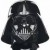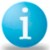# t value

Viewing 9 posts - 1 through 9 (of 9 total)
• Author
Posts
• #40019

what is t value / t statistics.How it is significant when we do a 2t test and deciding based on P value?
How to calculate a t value for a given degrees of freedom and alpha value?

0
#123084Ken Feldman
Participant

There are two t values that you are interested in when doing a two sample t test.  The first is the critical t which is based upon your degrees of freedom and alpha and the other is the calculated t which is calculated from your data.  But, there are two of these calculated t values depending on whether you have equal or unequal variances associated with your two sets of data.  In the old days you would look up the critical t in a table and then compare to your calculated t and then make a reject/don’t reject decision.  Nowadays, most programs calculate the p-value which is the area beyond your calculated t and you compare that to your selected alpha to make a reject/don’t reject decision.  You can do a Google search to get the exact formulas if you want them or some kind hearted poster can post them all including the t table.

0
#123112George Chynoweth
Participant

A t-value is actually a standard deviation score (this might give you a hint as to how it might be calculated) based on the t-distribution. The t-Test is useful if you have a small number of observations and only one or two groups of observations with which to conduct your hypothesis testing. When the number of observations (in each group) exceed 30, the t-distribution starts to look like the normal distribution, and the analysis of variance (ANOVA) becomes the preferred test. As Darth mentions, you calculate the t-value from your data (formula in every stat book) and compare it to a table of t-values. The tabled values are the critical values for specific Probabilites (.1, .05, .01, etc.) and degrees of freedom. If your calculated value is larger than the critical value, the result is said to be statistically significant at that Probability. You, the researcher, select the level of Probability based on your hypothesis.Note: If you are conducting a dependent samples t-Test (one group measured at two different points in time), it is important that the serial observations are not correlated with each other, as this will change the critical t-values. Tests of hypothesis are very sensitive to “autocorrelation”. By comparison, this issue is much more important than equality of variances.

0
#123305

Thanks george chynoweth and darth,
What is the relation between p value and t value.
How to calculate p value? how it is being done by minitab.

0
#123318

anbu,The p-values in the ANOVA table are calculated the same for GLM and ANOVA. The p-values from the ANOVA table are (1 – cdf for the F).

Go to File > Open Worksheet and select EXH_AOV.MTW. Go to Stat > ANOVA > Balanced ANOVA. Enter ‘Zooplankton’ as the response. For the model, enter
Supplement Lake Supplement*Lake

Click OK.

The output will look like the following.

ANOVA: Zooplankton versus Supplement, Lake Open Worksheet and select EXH_AOV.MTW. Go to Stat > ANOVA > Balanced ANOVA. Enter ‘Zooplankton’ as the response. For the model, enter
Supplement Lake Supplement*Lake

Click OK.

The output will look like the following.

ANOVA: Zooplankton versus Supplement, Lake Open Worksheet and select EXH_AOV.MTW. Go to Stat > ANOVA > Balanced ANOVA. Enter ‘Zooplankton’ as the response. For the model, enter
Supplement Lake Supplement*Lake

Click OK.

The output will look like the following.

ANOVA: Zooplankton versus Supplement, Lake ANOVA > Balanced ANOVA. Enter ‘Zooplankton’ as the response. For the model, enter
Supplement Lake Supplement*Lake

Click OK.

The output will look like the following.

ANOVA: Zooplankton versus Supplement, Lake ANOVA > Balanced ANOVA. Enter ‘Zooplankton’ as the response. For the model, enter
Supplement Lake Supplement*Lake

Click OK.

The output will look like the following.

ANOVA: Zooplankton versus Supplement, Lake Balanced ANOVA. Enter ‘Zooplankton’ as the response. For the model, enter
Supplement Lake Supplement*Lake

Click OK.

The output will look like the following.

ANOVA: Zooplankton versus Supplement, Lake Balanced ANOVA. Enter ‘Zooplankton’ as the response. For the model, enter
Supplement Lake Supplement*Lake

Click OK.

The output will look like the following.

ANOVA: Zooplankton versus Supplement, Lake Factor Type Levels Values
Supplement fixed 3 1, 2, 3
Lake fixed 2 Dennison, Rose

Analysis of Variance for Zooplankton

Source DF SS MS F P
Supplement 2 1918.5 959.3 9.25 0.015
Lake 1 21.3 21.3 0.21 0.666
Supplement*Lake 2 561.2 280.6 2.71 0.145
Error 6 622.0 103.7
Total 11 3123.0

S = 10.1817 R-Sq = 80.08% R-Sq(adj) = 63.49%

To calculate the p-value for Supplement from the simple model above:
Go to Calc > Probabilities Distributions > F… “Click on Cumulative probability”.
For “numerator degrees of freedom:”, enter the degrees of freedom for Supplement, which is 2.
For “denominator degrees of freedom:”, enter the degrees of freedom for the error term, which is 6
Click on “Input constant:”. In the box to the right, enter the numerical value of F for the factor, which is 9.25. Click OK.
The output will look like

Cumulative Distribution Function

F distribution with 2 DF in numerator and 6 DF in denominator
x P( X Probabilities Distributions > F… “Click on Cumulative probability”.
For “numerator degrees of freedom:”, enter the degrees of freedom for Supplement, which is 2.
For “denominator degrees of freedom:”, enter the degrees of freedom for the error term, which is 6
Click on “Input constant:”. In the box to the right, enter the numerical value of F for the factor, which is 9.25. Click OK.
The output will look like

Cumulative Distribution Function

F distribution with 2 DF in numerator and 6 DF in denominator
x P( X Probabilities Distributions > F… “Click on Cumulative probability”.
For “numerator degrees of freedom:”, enter the degrees of freedom for Supplement, which is 2.
For “denominator degrees of freedom:”, enter the degrees of freedom for the error term, which is 6
Click on “Input constant:”. In the box to the right, enter the numerical value of F for the factor, which is 9.25. Click OK.
The output will look like

Cumulative Distribution Function

F distribution with 2 DF in numerator and 6 DF in denominator
x P( X F… “Click on Cumulative probability”.
For “numerator degrees of freedom:”, enter the degrees of freedom for Supplement, which is 2.
For “denominator degrees of freedom:”, enter the degrees of freedom for the error term, which is 6
Click on “Input constant:”. In the box to the right, enter the numerical value of F for the factor, which is 9.25. Click OK.
The output will look like

Cumulative Distribution Function

F distribution with 2 DF in numerator and 6 DF in denominator
x P( X F… “Click on Cumulative probability”.
For “numerator degrees of freedom:”, enter the degrees of freedom for Supplement, which is 2.
For “denominator degrees of freedom:”, enter the degrees of freedom for the error term, which is 6
Click on “Input constant:”. In the box to the right, enter the numerical value of F for the factor, which is 9.25. Click OK.
The output will look like

Cumulative Distribution Function

F distribution with 2 DF in numerator and 6 DF in denominator
x P( X

0
#123329George Chynoweth
Participant

Hi Anbu,Don’t know about minitab.There are a number of articles & other resources on this site which can answer these basic questions. I encourage you to start reading.

0
#174942Saima Eman
Member

Q1. Why do we need a t value when we have a p value to decide whether the differences are significant or not?
Q2. Why do we use independent samples t test even when observations exceed 100 and there is no problem with it?

0
#174944Ken Feldman
Participant

A1.  You don’t but it is cool to know in case you want to verify the old fashioned way in the tables
A2.  Still some slight difference between the Z and t even at 100.  It is likely you will get the same answer regardless of which you use.  T approaches Z at some point so you don’t lose anything by using a t.

0
#174950Saima Eman
Member

Thank you for the answers Darth. I got the answer to first question which I think is that we want to see standard deviations, I mean significance in terms of standard scores; so we see t and when we want to know the percentages we see p value. Its just like frequencies and percentages….
However, i cannot find information about Std. Error of difference, its purpose, etc…

0
Viewing 9 posts - 1 through 9 (of 9 total)

The forum ‘General’ is closed to new topics and replies.